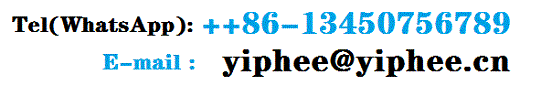Products

50Kg induction furnace

Rated input voltageInput power frequencyNumber of input power phasesRated input currentRated output ···

100Kg induction melting ···

Rated input voltageInput power frequencyNumber of input power phasesRated input currentRated output ···

150Kg induction furnace

Rated input voltageInput power frequencyNumber of input power phasesRated input currentRated output···

200Kg induction furnace

Input power frequencyNumber of input power phasesRated input currentRated output powerRated output f···

250Kg induction furnace

Rated input voltageInput power frequencyNumber of input power phasesRated input currentRated output ···

350kg capacity medium fr···

Rated input voltageInput power frequencyNumber of input power phasesRated input currentRated output ···

500 kg capacity inductio···

Rated input voltageInput power frequencyNumber of input power phasesRated input currentRated output ···

Medium frequency inducti···

Rated input voltageInput power frequencyNumber of input power phasesRated input currentRated output ···